Next: *DASHPOT Up: Input deck format Previous: *CYCLIC SYMMETRY MODEL   Contents

## *DAMPING

Keyword type: model definition, if structural damping: material

This card is used to define Rayleigh damping for implicit dynamic calculations (*DYNAMIC) and structural damping for steady state dynamics calculations (*STEADY STATE DYNAMICS).

For Rayleigh damping there are two required parameters: ALPHA and BETA.

Rayleigh damping is applied in a global way, i.e. the damping matrix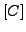is taken to be a linear combination of the stiffness matrix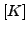and the mass matrix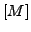: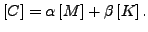(467)

The damping force satisfies: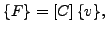(468)

where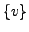is the velocity vector. For Rayleigh damping only one *DAMPING card can be used in the input deck. It applies to the whole model.

For structural damping the damping is a material characteristic. Each material can have its own damping value. There is one required parameter STRUCTURAL, defining the value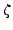of the damping. For structural damping the element damping force is displacement dependent and satisfies: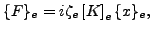(469)

where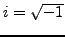,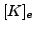is the element stiffness matrix, and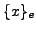is the element displacement vector.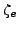is the structural damping value for the material of element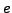(default is zero). The global damping force is assembled from the element damping forces.

First line:

• *DAMPING
• Enter ALPHA and BETA and their values for Rayleigh damping or STRUCTURAL and its value for structural damping.

Example:

*DAMPING,ALPHA=5000.,BETA=2.e-3


indicates that a damping matrix is created by multiplying the mass matrix with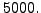and adding it to the stiffness matrix multiplied by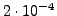Example:

*DAMPING,STRUCTURAL=0.03


defines a structural damping value of 0.03 (3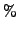). This card must be part of a material description.

Example files: beamimpdy1, beamimpdy2.Next: *DASHPOT Up: Input deck format Previous: *CYCLIC SYMMETRY MODEL   Contents
guido dhondt 2018-12-15